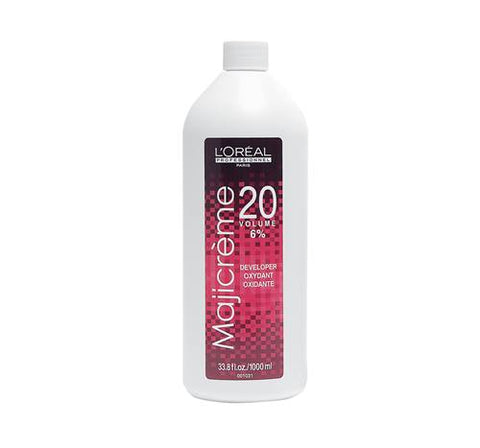Surat, Gujarat
6 hours ago

# How to Mix Developer

## At some point or another, most hair colour technicians will need to mix together a couple of different developers in order get the perfect volume that they need.For example, you probably will not have 25 volume sitting around, so naturally, you will mix half of 20 volume and half of 30 volume in order to make 25 volume.

And say you need to make 20 volume, but you only have 40 volume lying around. You would naturally mix 1 ounce of 40 volume and 1 ounce of cream conditioner (or some other neutral mixture) to get a solution that averages out to 20 volume.

This is pretty straight forward and something you’re probably used to doing in the salon anyways.

But….

On your Ontario Hairstylist licensing exam, you might get a question that looks something like this:

Which of the following combinations would create 30 volume developer?

1. 20 + 10 + 20
2. 50 + 20 + 20
3. 10 + 40 + 20
4. 40 + 40 + 20

Pretty confusing if you’ve never even seen 50 volume and if you’ve never had to mix developer this way.

So, here’s a formula I’ve developed that you can use to tackle a question like this on your hair license exam:

(X1 + X2 + X3 + …) / Y = Z

Where X1 , X2 , X3….. represent 1 oz of any volume of developer

Y represents the total number of ounces of developer

Z represents the final volume of the resulting developer

So based on this formula, the correct answer to the question above is B.

Why?

In the question above, assume that each number you see represents 1 oz (ounce) of developer, so that there are 3 equal parts of 3 different developers.

Since you need 30 volume, and there are 3 ounces of developer in each of the possible answers, it means that the total volume of all of those 3 ounces combined when added together must equal to 30 when it is divided into 3 equal parts.

What divided by 3 will equal to 30?....let’s do some reverse math à 3 x 30 = 90.

Therefore, the correct answer in the question above must add up to 90 because 90 ÷ 3 = 30.

Only option B in the answer above adds up to 90. 50 volume + 20 volume + 20 volume = 90 volume.

90 volume ÷ into 3 equal parts = 30 volume.

We are simply averaging out the level of the volume when 3 equal portions of different volumes are combined.

Another Example:

You ran out of 20 volume and you only have 40 and 10 volume available. How could you create 20 volume?

You must mix:

1 part 40 + 1 part 10 + 1 part 10

Why:

(40 + 10 + 10) ÷ 3 = 20

Makes sense, right?

Well, I hope I have simplified what may have initially seemed like a tricky or confusing exam question.

Best of luck on your exam prep and studying!

More study notes and questions for practice are available here.

Source:

https://www.hairfinder.com/hair4/mix-peroxide-formula.htm

>